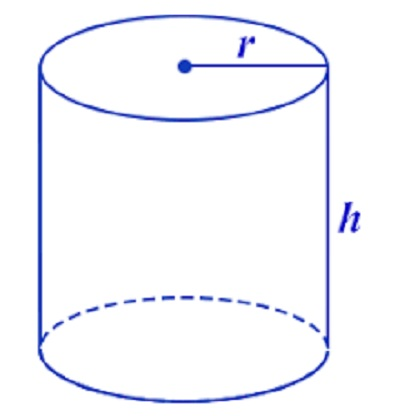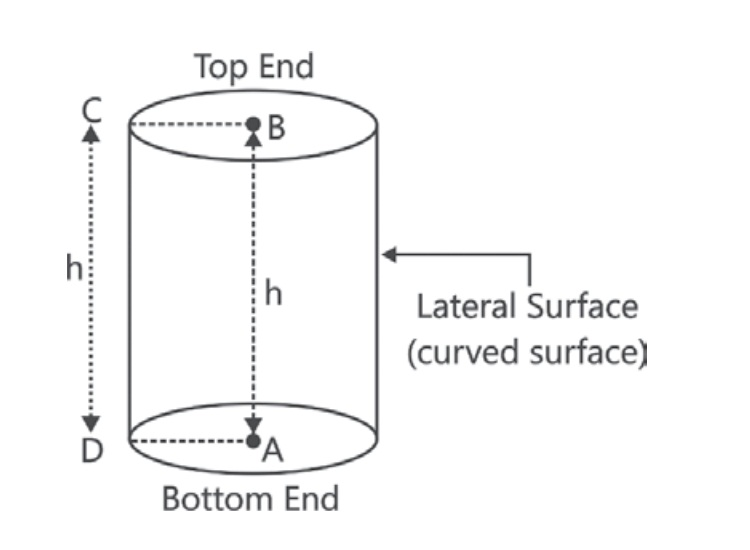# Cylinder Area Calculator

In mathematical terms, a right circular cylinder is a solid generated by the revolution of a rectangle about its sides. Solids like circular pillars, circular pipes, circular pencils, measuring jars, road rollers and gas cylinders, etc., are said to be in cylindrical shape. Cylinder area calculator is an online tool to calculate surface area, lateral surface and base surface area and the volume of a cylinder. Enter the radius (r) and height (h) to calculate area and volume of cylinder.## What is a Cylinder ?

Let the rectangle ABCD revolve about its side AB, so as to describe a right circular cylinder as shown in the figure.You must have observed that the cross-sections of a right circular cylinder are circles congruent and parallel to each other.

The following are definitions of some terms related to a right circular cylinder:

• The radius of any circular end is called the radius of the right circular cylinder. Thus, in the given figure, AD as well as BC is a radius of the cylinder.
• The line joining the centres of circular ends of the cylinder, is called the axis of the right circular cylinder. In the above figure, the line AB is the axis of the cylinder. Clearly, the axis is perpendicular to the circular ends.
• The length of the axis of the cylinder is called the height or length of the cylinder.
• The curved surface joining the two bases of a right circular cylinder is called its lateral surface.

Note: If the line joining the centres of circular ends of a cylinder is not perpendicular to the circular ends, or if the ends of the cylinder are not proper circles then the cylinder is not a right circular cylinder.

## Surface Area and Volume of a Cylinder Formula

For a right circular cylinder of radius = r units and height = h units, we have:

Area of each circular end : πr2 sq. units.

Curved (Lateral) Surface Area : 2πrh sq. units.

Total Surface Area = Curved Surface Area + Area of two circular ends

Therefore, Total Surface Area of Cylinder : 2πrh+2πr2 sq. units.

Volume of Cylinder : πr2h

The above formulae are applicable to solid cylinders only